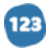# Heat Exchangers

analytical Essay
1046 words
1046 wordsEnergy Balances

From an energy balance around a heat exchanger, it is clear that the heat duties

calculated on the hot and cold sides should be equivalent. The data obtained

throughout this experiment were consistent with this assertion. This is shown in Figure

4.4. In the figure, it can be seen that the heat duties on the hot and cold sides are

equivalent (within experimental error) for all runs of the finned, double-pipe heat

exchanger. The energy balances for the runs in the other exchangers are similarly

satisfied, as seen in Tables 4.1 and 4.2.

The fact that the energy balances are satisfied allows confidence to be placed in

the data obtained throughout the experiment. This, in turn, allows confidence to be

placed in the scale-up.

Analysis of the Wilson Plots

The Wilson Plot method makes use of the fact that when the velocity of the hot

fluid in a heat exchanger is varied, the only resistance to heat transfer that changes is

the fluid coefficient, h. There are empirical correlations to determine the Nusselt number

as a function of the Reynolds number and the Prandtl number. From these correlations,

it can be shown that h v0.8 for sufficiently high fluid velocity. As such, it follows that a

plot of 1/U versus 1/v0.8 should be linear.

As seen in Figures 4.1, 4.2 and 4.3, the data obtained in this experiment are

consistent with this theory. Plots of 1/U versus 1/v0.8 for all exchangers tested in this

experiment were remarkably linear. This is evident from the coefficient of determination

(R2) values of the best fit lines. The R2 value for the high cold flow rate data in the plate

heat exchanger, for example, was 0.998. This means that 99.8 percent of the variation

in the data is accounted for in the lin...

... middle of paper ...

...ndations for Future Study

There are a few areas for improvements for future experiments. In future

experiments, the piping system should be better studied so that valves can be set up

more quickly. This would allow for more runs to be accomplished.

In addition, the cold tank should not be filled to capacity in future experiments

because the cold flow rates were not stable when the tank was full. Instead, filling the

tank to about half capacity would produce more stable cold flows, and reduce time until

Conclusions

Overall, this experiment successfully examined three different types of heat

exchangers for their feasibility for a scaled up process. Based on the overall heat

transfer coefficients determined experimentally, the plate heat exchanger provides the

most efficient heat transfer and thus should be selected for scale up.

#### In this essay, the author

• Explains that from an energy balance around a heat exchanger, it is clear that the heat duties.
• Illustrates the heat duties on the hot and cold sides of the figure.
• Explains that it can be shown that h v0.8 for sufficiently high fluid velocity.

•